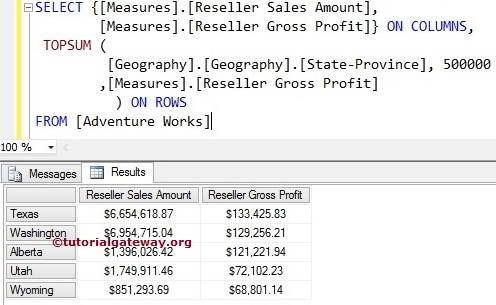# MDX TOPSUM Function

The MDX TopSum function will sort the given data in the descending order. Then selects the required number of records from the sorted data whose total is at least equal to the specified value.

## MDX TopSum Function Syntax

The basic syntax of the MDX TopSum in Multidimensional Expression is as shown below:

`TOPSUM (Set_Expression, Value, Numeric_Expression)`
• Set_Expression: Any Multidimensional Expression or attributes on which you want to check.
• Value: Please provide the Value. The MDX TOPSUM function will retrieve the records whose total is at least this Value.
• Numeric_Expression: Any Multidimensional Expression or measure. The TopSum function will use this Numeric_Expression to sort the data in descending order and then picks the number of records required to match the Value.

Write the MDX TopSum function to extract the States from Geography Dimension (present in the Adventure Works Cube), whose total is at least equal to the user given Value. For this, we are going to use the below-shown data.

## MDX TopSum Function Example

The following MDX TOPSUM query returns all the states present in the Geography Dimension, who are contributing Sale of 40000000 of total Reseller Sales Amount.

```SELECT {[Measures].[Reseller Sales Amount]} ON COLUMNS,
TOPSUM (
[Geography].[Geography].[State-Province], 40000000
,[Measures].[Reseller Sales Amount]
) ON ROWS

In the above MDX Query, We selected the [Reseller Sales Amount] Measure on the Columns and [State Province] column present in the geography Dimension on Rows. Next, TopSum function will sort the data in the descending order using Measures.[Reseller Sales Amount] and then add the Sales amount of each state until it reaches 40000000.If you observe the above screenshot, If you total the Sales amount of above-displayed states, they approximately equal to 40243296.5. It means greater than what we required.

## MDX TopSum Function Example 2

In this example, We are going to use two measures ([Measures].[Reseller Sales Amount] [Measures].[Reseller Gross Profit]).

```SELECT {[Measures].[Reseller Sales Amount],
[Measures].[Reseller Gross Profit]} ON COLUMNS,
TOPSUM (
[Geography].[Geography].[State-Province], 500000
,[Measures].[Reseller Gross Profit]
) ON ROWS